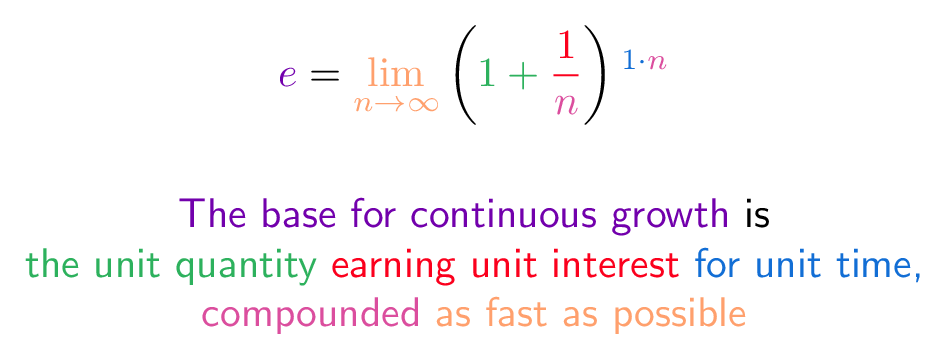# # E (Mathematical Constant)

## # Colorized Definition\newcommand{\growth}{\color{c1}}
\newcommand{\unitQuantity}{\color{c2}}
\newcommand{\unitInterest}{\color{c3}}
\newcommand{\unitTime}{\color{c4}}
\newcommand{\perfectly}{\color{c5}}
\newcommand{\compounded}{\color{c6}}

$$\growth e \plain = \perfectly \lim_{n\to\infty} \plain \left( \unitQuantity 1 + \unitInterest \frac{1}{\compounded n} \plain \right) \unitTime^{1 \cdot \compounded n}$$

\growth       The base for continuous growth
\plain        is
\\
\unitQuantity the unit quantity
\unitInterest earning unit interest
\unitTime     for unit time,
\\
\compounded   compounded
\perfectly    as fast as possible


## # Plain English

• What is e? A constant (2.718...) representing continuous unit growth: the unit quantity (1.0), continuously growing the unit rate (100%), for unit time (1 period).

• Why's e special? All circles are the unit circle, scaled up. All continuously growing systems are e^{rt}, scaled to some rate and time.

• When should I use e? Use e^{rt} for things that change constantly (radioactive decay, populations). For growth based on discrete intervals (interest payments, combinatorics), (1 + rate)^{time} is a better model.